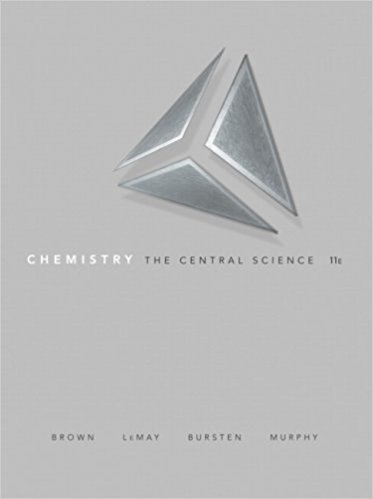> > > > Problem 7.34

# Consider the isoelectronic ions o- and K + (a) Which ion is smaller (b) Use Equation 7.1## Problem 7.34 Chapter 7

Chemistry: The Central Science | 11th Edition

• 2901 Step-by-step solutions solved by professors and subject experts
• Get 24/7 help from StudySoup virtual teaching assistantsChemistry: The Central Science | 11th Edition

4 5 0 255 Reviews
30
0
Problem 7.34

Consider the isoelectronic ions o- and K + (a) Which ion is smaller? (b) Use Equation 7.1 and assuming that core electrons contribute 1.00 and valence electrons contribute nothing to the screening constant, S, calculate Zeff for these two ions. (c) Repeat this calculation using Slater's rules to estimate the screening constant, S. (d) For isoelectronic ions how are effective nuclear charge and ionic radius related?

Step-by-Step Solution:
Step 1 of 3

March 22, 2016 - trascription, translation - 45 monosaccarides - amino acids, acid-base structure n = 4 monosaccarides diastereomers - not mirror images Stereochemical Relationships of Ketoses Psyco Fruits Sort Tags ﬁve membered (furanose) rings six membered (pyranose) rings above - Beta - better up be comfortable going from...

Step 2 of 3

Step 3 of 3

##### ISBN: 9780136006176

The answer to “Consider the isoelectronic ions o- and K + (a) Which ion is smaller? (b) Use Equation 7.1 and assuming that core electrons contribute 1.00 and valence electrons contribute nothing to the screening constant, S, calculate Zeff for these two ions. (c) Repeat this calculation using Slater's rules to estimate the screening constant, S. (d) For isoelectronic ions how are effective nuclear charge and ionic radius related?” is broken down into a number of easy to follow steps, and 66 words. This textbook survival guide was created for the textbook: Chemistry: The Central Science , edition: 11. The full step-by-step solution to problem: 7.34 from chapter: 7 was answered by , our top Chemistry solution expert on 03/09/18, 07:03PM. Since the solution to 7.34 from 7 chapter was answered, more than 216 students have viewed the full step-by-step answer. Chemistry: The Central Science was written by and is associated to the ISBN: 9780136006176. This full solution covers the following key subjects: . This expansive textbook survival guide covers 25 chapters, and 2360 solutions.

Unlock Textbook Solution

Consider the isoelectronic ions o- and K + (a) Which ion is smaller (b) Use Equation 7.1

×
Get Full Access to Chemistry: The Central Science - 11 Edition - Chapter 7 - Problem 7.34

Get Full Access to Chemistry: The Central Science - 11 Edition - Chapter 7 - Problem 7.34

I don't want to reset my password

Need help? Contact support

Need an Account? Is not associated with an account
We're here to help